search UMD   This sitePrinciple of Work and Energy. If the applet doesnt load, you may need to install the appropriate plugins, a link to the plugins can be found
 User’s Guide This Simulation covers: A free body diagram of the body when it is located at an intermediate point along the path, in order to account for all the forces and couples which do work on the body as it moves along the path. The principle of Work and Energy is used here to solve the kinetics problem as this involves velocity, force and displacement. Kinetic Energy:  Since the disk rotates about a fixed axis, the Kinetic Energy (T) can be computed using: T          =          ½ Io * ω2 , where moment of inertia (Io) is Io          =          ½ m * r2 Work (Free-Body Diagram):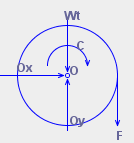As shown in diagram, the pin reactions Ox and Oy and the weight do not work, since they are not displaced. The couple moment C, having a constant magnitude, does positive work            UM       =          M * θ As the disk rotates through a clockwise angle of θ rad, and the constant force F does positive work    UFc       =          Fs As the cord moves downward  s         =          θ *r Principle of Work and Energy:  {T1}    +          {∑U1-2}           =         {T2} {T1}    +          {M * θ + Fs}  =          {T2}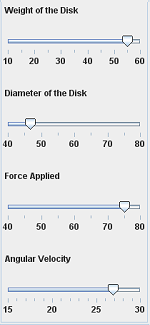Various inputs can be given using sliders on this panel. Also, the final angular velocity can be specified with the slider at the bottom.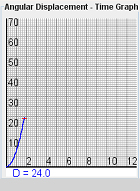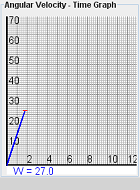The behavior of Angular Velocity and Displacement with respect to time can be viewed through the graphs.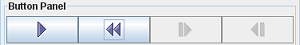Animation can be played, paused, reset through the Animation Control Buttons.This is a sample screen with the body slide down the screen.